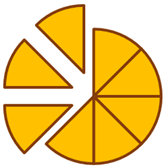200 students

## Mathematics

Mathematics enhances our analytical, calculative, and estimating skills, needed throughout the daily life.  It is the science of numbers and their operations, interrelations, combinations, generalizations, and abstractions and of space configurations and their structure, measurement, transformations, and generalizations. Algebra, arithmetic, calculus, geometry, and trigonometry are branches of mathematics.

Here, children can opt for Mathematics course along with the other subjects. This course is spread over all the grades in our school and advances along with the following areas.

• Basic, Advanced, Pure and Applied mathematics.
• Mathematical Methodology, Statements, and General concepts.
• Mathematical objects, Equations.
• Integrals, Derivatives, Calculus.

So let’s get started. I’m sure you’ll love it.You can pay for this course using a debit or credit card:
We also honour bank transfers: